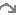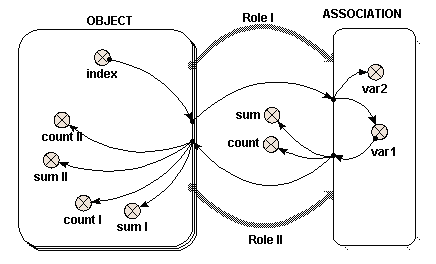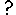#Two role arrows from the same submodel

The most common use of the role arrow is (unfortunately) the most difficult to visualise. In this case, two different instances of the same object play two different roles in an association. One might think of this as being like each instance having all the other instances nested within it.

In the case of the single role arrow, or the case of two role arrows from two objects, the role itself was not an important element. The role arrow represented graphically the nesting arrangement. In this case however, the names of the two different role arrows are the only means of distinguishing the instances of the single object within the association submodel.

The following model diagram illustrates a simple example of the use of the association submodel in this way. There is an "Object" submodel, which has three instances. Two role arrows, "Role I" and "Role II", link "Object" to the "Association" submodel.In this case, nine instances of "Association" are created, one for each possible pair of instances of "Object". Inside "Association" are two variables "var1" and "var2". Each of these receives a single influence arrow from the "index" variable in "Object", BUT, look at the list of parameters in the equation dialogue box, and two are given. These two parameters refer to the "index" variable in each of the members of the pair of instances of "Object" that generate this instance of "Association".

A unique local name for each parameter is automatically generated, but to avoid confusion, right click on each local name to rename it indexI and indexII, for "Value(s) of ../Object/index (from Object in Role I)" and "Value(s) of ../Object/index (from Object in Role II)" respectively. In this example, "var1" is given the expression "indexI" and "var2" is given the expression "indexII".

If this were represented as a table, it would look like this:

 Object in Role I "indexI" = 1 Object in Role I "indexI" = 2 Object in Role I "indexI" = 3 Object in Role II "indexII" = 1 var1 = 1 var2 = 1 var1 = 2 var2 = 1 var1 = 3 var2 = 1 Object in Role II "indexII" = 2 var1 = 1 var2 = 2 var1 = 2 var2 = 2 var1 = 3 var2 = 2 Object in Role II "indexII" = 3 var1 = 1 var2 = 3 var1 = 2 var2 = 3 var1 = 3 var2 = 3

So far, the results are rather similar to those where two objects are involved. This is also true of what follows, though it is less obvious. To understand how "var1" is represented, six influence arrows are drawn from it to different parts of the diagram. (Symmetrical results are generated using "var2", though these are not given here.)

Inside "Object", "var1" again appears as two distinct parameters, distinguished by the name of the role arrow. Alter the local names to "var1_I" and "var1_II" for "Value(s) of ../Association/var1 (to Object in Role I)" and "Value(s) of ../Association/var1 (to Object in Role II)" respectively, as before. Look at the full nine-instance table above to determine the values of "var1_I" and "var1_II", by reading across (for "Role II") or down (for "Role I") the relevant line.
 OBJECT Values of var1 for role I sum count values of var1 for role II sum count Instance 1 {1 1 1} 3 3 {1 2 3} 6 3 Instance 2 {2 2 2} 6 3 {1 2 3} 6 3 Instance 3 {3 3 3} 9 3 {1 2 3} 6 3
What's going on here is that, since the values of "var1" in each instance of the association submodel are set from the instance of the "Object" in "Role I", then the values going to the "Object" in Role I" are the same as what came from it for each instance. On the other hand, the values going to the "object" in "Role II" are all different, since the associations with that instance in "Role II" each have a different instance in "Role I". This is how the association enables values to be passed selectively between different instances of a submodel -- by taking them to the association in one role and bringing them back to the base instance in another. The values would be passed in the reverse direction if those from "var2" (which are set from "objects" in "Role II") are passed back to those in "Role I".

Outside "Object", "var1" has the value {1 1 1 2 2 2 3 3 3} and thus count({var1}) is 9 and sum({var1}) is 18. Note that values from all nine instances of "Association" are received by elements outside "Object".

Thecondition symbol can be used inside "Association" to permit fewer instances than the square of the number of instances of "Object" to be generated, and thus pass values between base instances selectively.

Inside the association submodel, the index(x) function returns the index of each of the members of the pair of instances that generate this instance of "Association" with x = 1 for "Dimension 1 of Object (3) in Role II for Association" and x = 2 for "Dimension 1 of Object (3) in Role I for Association". To identify which index refers to the use of which role arrow, see the indices panel on the Parameters tab of the equation dialogue box.

Note that the "(3)" is the size of the given dimension. Influences from other elements can also be used in the Boolean expression for the condition symbol.